# 使用 MLflow 和 Azure 机器学习跟踪 ML 模型

MLflow 是一个开放源代码库，用于管理机器学习试验的生命周期。 MLFlow 跟踪是 MLflow 的一个组件，它可以记录和跟踪训练运行指标及模型项目，无论试验环境是在本地计算机上、远程计算目标上、虚拟机上，还是在 Azure Databricks 群集上。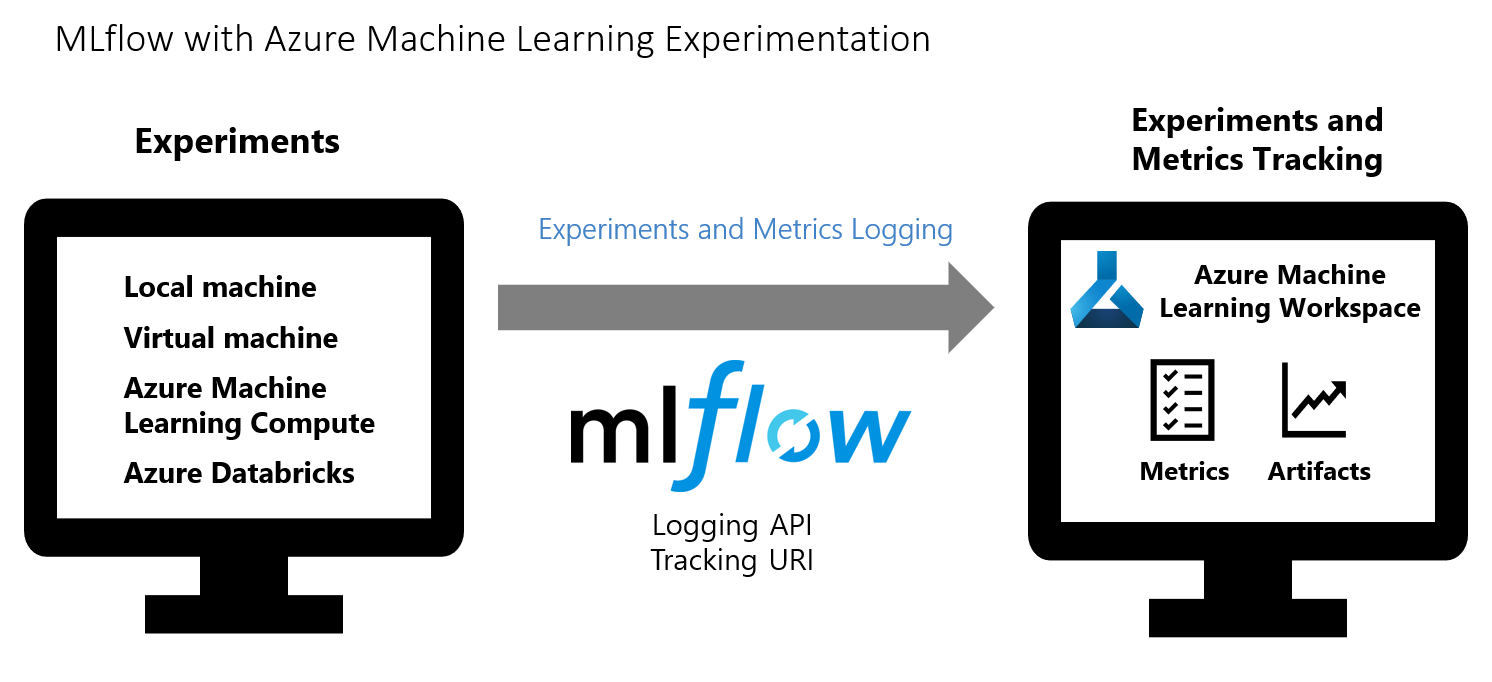## 跟踪本地运行

``````import mlflow
from azureml.core import Workspace

ws = Workspace.from_config()

mlflow.set_tracking_uri(ws.get_mlflow_tracking_uri())
``````

``````experiment_name = 'experiment_with_mlflow'
mlflow.set_experiment(experiment_name)

with mlflow.start_run():
mlflow.log_metric('alpha', 0.03)
``````

## 跟踪远程运行

``````name: sklearn-example
dependencies:
- python=3.6.2
- scikit-learn
- matplotlib
- numpy
- pip:
- azureml-mlflow
- mlflow
- numpy
``````

``````import mlflow

with mlflow.start_run():
mlflow.log_metric('example', 1.23)
``````

``````run = exp.submit(src)
``````

## 查看工作区中的指标和项目

MLflow 记录的指标和项目保存在工作区中。 若要随时查看它们，请在 Azure 机器学习工作室中导航到你的工作区，并在该工作区中按名称找到试验。 或运行以下代码。

``````run.get_metrics()
``````

## 管理模型

1. 运行完成后，调用 `register_model()` 方法。

``````# the model folder produced from the run is registered. This includes the MLmodel file, model.pkl and the conda.yaml.
run.register_model(model_name = 'my-model', model_path = 'model')
``````
2. 使用 Azure 机器学习工作室查看工作区中的已注册模型。

在以下示例中，已注册的模型 `my-model` 标记了 MLflow 跟踪元数据。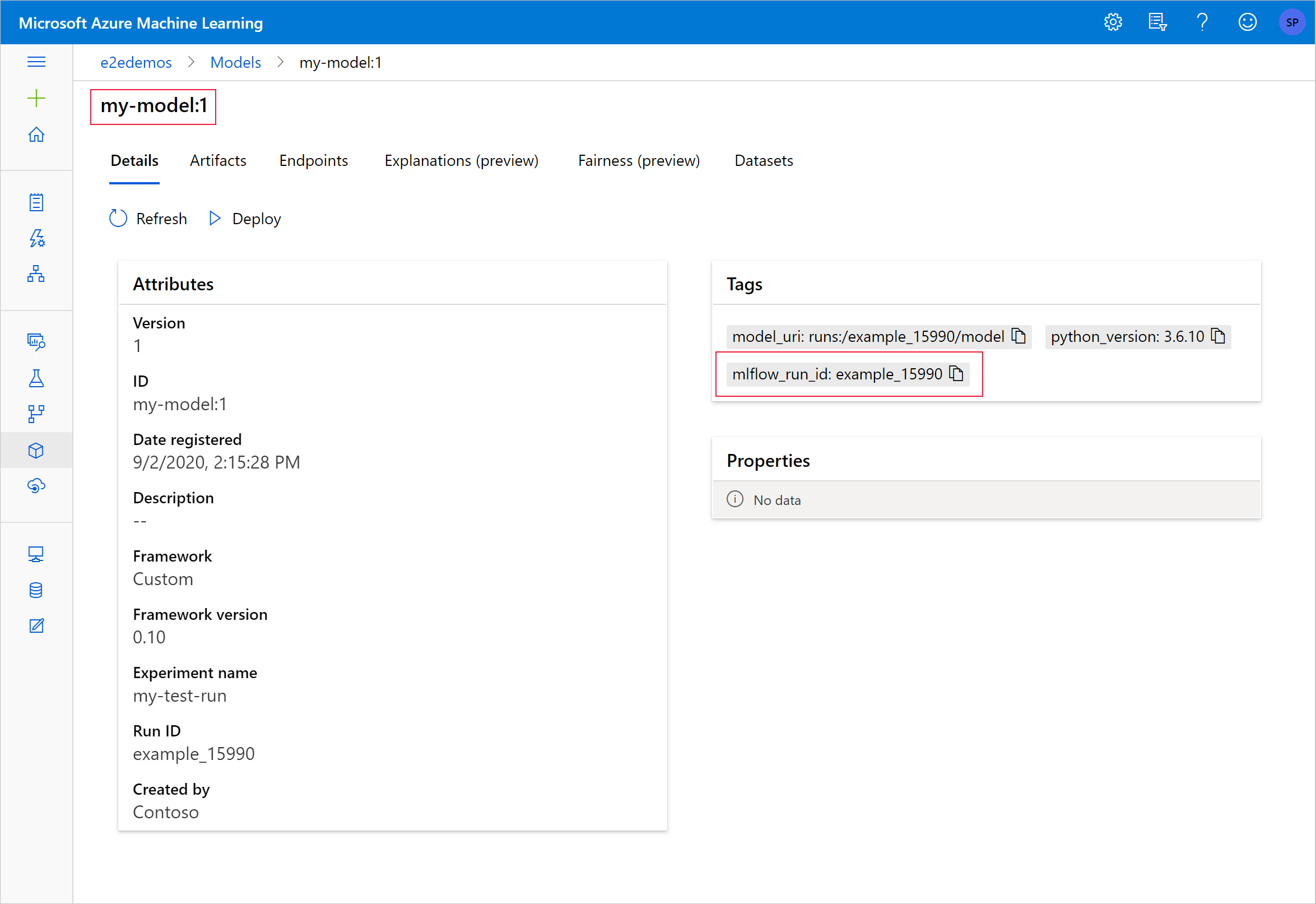3. 选择“项目”选项卡以查看与 MLflow 模型架构（conda.yaml、MLmodel 和 model.pkl）一致的所有模型文件。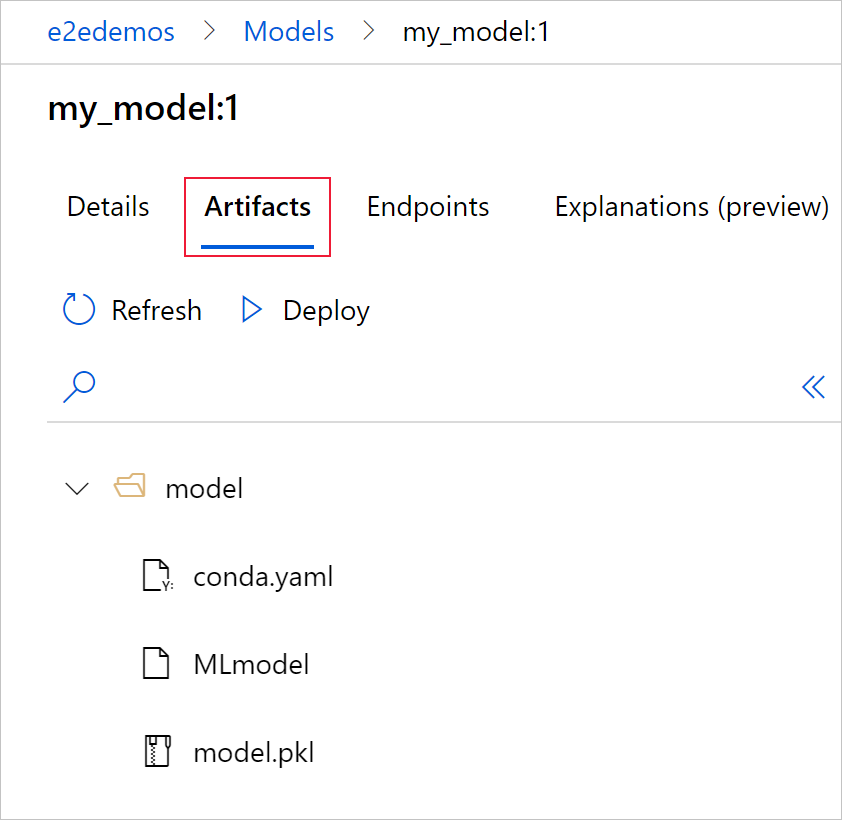4. 选择 MLmodel 以查看运行生成的 MLmodel 文件。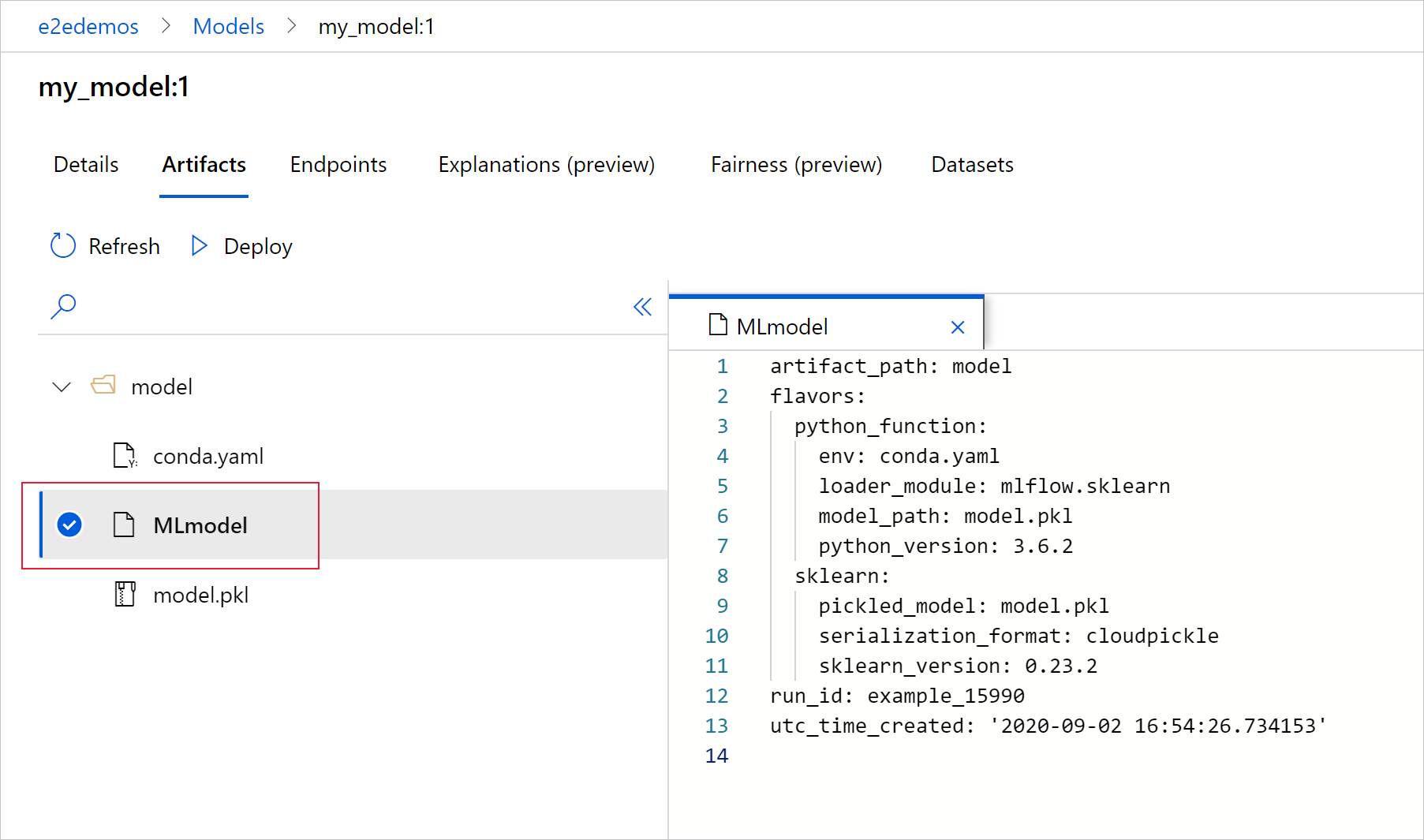## 清理资源

1. 在 Azure 门户中，选择最左侧的“资源组”。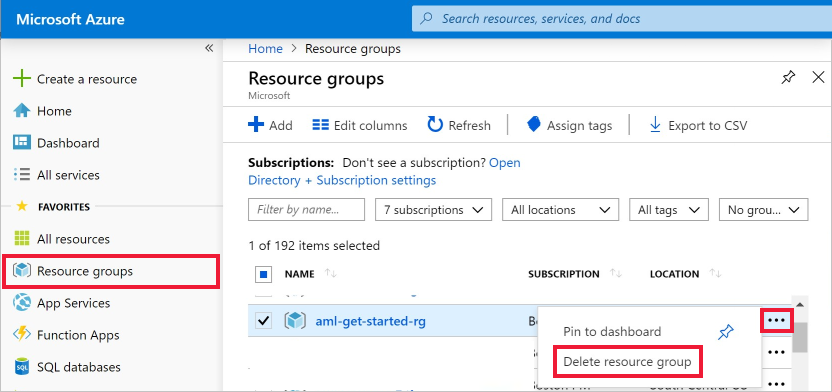2. 从列表中选择已创建的资源组。

3. 选择“删除资源组”。

4. 输入资源组名称。 然后选择“删除”。